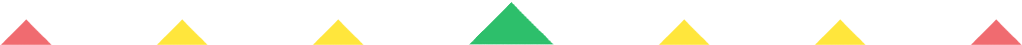Player 1
vs
Player 2
0:00
Opponent history
x
xCorrect
0
Wrong
0
Correct!
Wrong!
Help Sheet for

• 0 / 1 = 0
• 1 / 1 = 1
• 2 / 1 = 2
• 3 / 1 = 3
• 4 / 1 = 4
• 5 / 1 = 5
• 6 / 1 = 6
• 7 / 1 = 7
• 8 / 1 = 8
• 9 / 1 = 9
• 10 / 1 = 10
• 11 / 1 = 11
• 12 / 1 = 12

• 0 / 2 = 0
• 2 / 2 = 1
• 4 / 2 = 2
• 6 / 2 = 3
• 8 / 2 = 4
• 10 / 2 = 5
• 12 / 2 = 6
• 14 / 2 = 7
• 16 / 2 = 8
• 18 / 2 = 9
• 20 / 2 = 10
• 22 / 2 = 11
• 24 / 2 = 12

• 0 / 3 = 0
• 3 / 3 = 1
• 6 / 3 = 2
• 9 / 3 = 3
• 12 / 3 = 4
• 15 / 3 = 5
• 18 / 3 = 6
• 21 / 3 = 7
• 24 / 3 = 8
• 27 / 3 = 9
• 30 / 3 = 10
• 33 / 3 = 11
• 36 / 3 = 12

• 0 / 4 = 0
• 4 / 4 = 1
• 8 / 4 = 2
• 12 / 4 = 3
• 16 / 4 = 4
• 20 / 4 = 5
• 24 / 4 = 6
• 28 / 4 = 7
• 32 / 4 = 8
• 36 / 4 = 9
• 40 / 4 = 10
• 44 / 4 = 11
• 48 / 4 = 12

• 0 / 5 = 0
• 5 / 5 = 1
• 10 / 5 = 2
• 15 / 5 = 3
• 20 / 5 = 4
• 25 / 5 = 5
• 30 / 5 = 6
• 35 / 5 = 7
• 40 / 5 = 8
• 45 / 5 = 9
• 50 / 5 = 10
• 55 / 5 = 11
• 60 / 5 = 12

• 0 / 6 = 0
• 6 / 6 = 1
• 12 / 6 = 2
• 18 / 6 = 3
• 24 / 6 = 4
• 30 / 6 = 5
• 36 / 6 = 6
• 42 / 6 = 7
• 48 / 6 = 8
• 54 / 6 = 9
• 60 / 6 = 10
• 66 / 6 = 11
• 72 / 6 = 12

• 0 / 7 = 0
• 7 / 7 = 1
• 14 / 7 = 2
• 21 / 7 = 3
• 28 / 7 = 4
• 35 / 7 = 5
• 42 / 7 = 6
• 49 / 7 = 7
• 56 / 7 = 8
• 63 / 7 = 9
• 70 / 7 = 10
• 77 / 7 = 11
• 84 / 7 = 12

• 0 / 8 = 0
• 8 / 8 = 1
• 16 / 8 = 2
• 24 / 8 = 3
• 32 / 8 = 4
• 40 / 8 = 5
• 48 / 8 = 6
• 56 / 8 = 7
• 64 / 8 = 8
• 72 / 8 = 9
• 80 / 8 = 10
• 88 / 8 = 11
• 96 / 8 = 12

• 0 / 9 = 0
• 9 / 9 = 1
• 18 / 9 = 2
• 27 / 9 = 3
• 36 / 9 = 4
• 45 / 9 = 5
• 54 / 9 = 6
• 63 / 9 = 7
• 72 / 9 = 8
• 81 / 9 = 9
• 90 / 9 = 10
• 99 / 9 = 11
• 108 / 9 = 12

###Divide by 10

• 0 / 10 = 0
• 10 / 10 = 1
• 20 / 10 = 2
• 30 / 10 = 3
• 40 / 10 = 4
• 50 / 10 = 5
• 60 / 10 = 6
• 70 / 10 = 7
• 80 / 10 = 8
• 90 / 10 = 9
• 100 / 10 = 10
• 110 / 10 = 11
• 120 / 10 = 12

###Divide by 11

• 0 / 11 = 0
• 11 / 11 = 1
• 22 / 11 = 2
• 33 / 11 = 3
• 44 / 11 = 4
• 55 / 11 = 5
• 66 / 11 = 6
• 77 / 11 = 7
• 88 / 11 = 8
• 99 / 11 = 9
• 110 / 11 = 10
• 121 / 11 = 11
• 132 / 11 = 12

###Divide by 12

• 0 / 12 = 0
• 12 / 12 = 1
• 24 / 12 = 2
• 36 / 12 = 3
• 48 / 12 = 4
• 60 / 12 = 5
• 72 / 12 = 6
• 84 / 12 = 7
• 96 / 12 = 8
• 108 / 12 = 9
• 120 / 12 = 10
• 132 / 12 = 11
• 144 / 12 = 12